# ISEE Middle Level Math : How to find the probability of an outcome

## Example Questions

1 2 3 4 5 6 8 Next →

### Example Question #71 : How To Find The Probability Of An Outcome

If a class hasboys andgirls, what is the probability the teacher will call on a boy?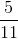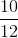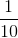Explanation:

To find the probability of an event, we will use the following formula: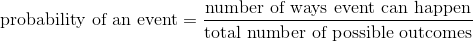Now, in the event of calling on a boy in the class, we can determine the number of ways the event can happen: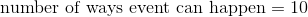because there are 10 boy students in the class.

To find the number of possible outcomes, we get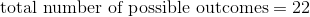because there are 22 total students the teacher could potentially call on.

Knowing all of this, we can substitute into the formula.  We get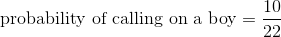Reduce: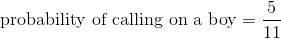Therefore, the probability of calling on a boy is.

1 2 3 4 5 6 8 Next →

### All ISEE Middle Level Math Resources